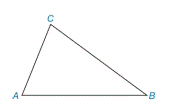Chapter 2.4, Problem 2EElementary Geometry For College St...

7th Edition
Alexander + 2 others
ISBN: 9781337614085

Solutions

Chapter
SectionElementary Geometry For College St...

7th Edition
Alexander + 2 others
ISBN: 9781337614085
Textbook Problem

In Exercise 1 to 4, refer to Δ A B C . On the basis of the information given, determine the measure of the remaining angle(s) of the triangle. m ∠ B = 39 ° and m ∠ C = 82 °To determine

To find:

The measure of A.

Explanation

Given:

The given statement is,

mB=39° and mC=82°

Figure (1)

Calculation:

In a triangle, the sum of the measures of the interior angles is 180°.

The given statement is,

mB=39° and mC=82°.

The sum of the measures of the interior angles is 180°

Still sussing out bartleby?

Check out a sample textbook solution.

See a sample solution

The Solution to Your Study Problems

Bartleby provides explanations to thousands of textbook problems written by our experts, many with advanced degrees!

Get Started

Solve the equations in Exercises 126. x+4x+1+x+43x=0

Finite Mathematics and Applied Calculus (MindTap Course List)

x3a+b3

Applied Calculus for the Managerial, Life, and Social Sciences: A Brief Approach

Differentiate. f(t)=t3t3

Calculus: Early Transcendentals

True or False: converges.

Study Guide for Stewart's Multivariable Calculus, 8th

True or False: Every series must do one of these: converge absolutely, converge conditionally, or diverge.

Study Guide for Stewart's Single Variable Calculus: Early Transcendentals, 8th